﻿
It is the top of the page.

#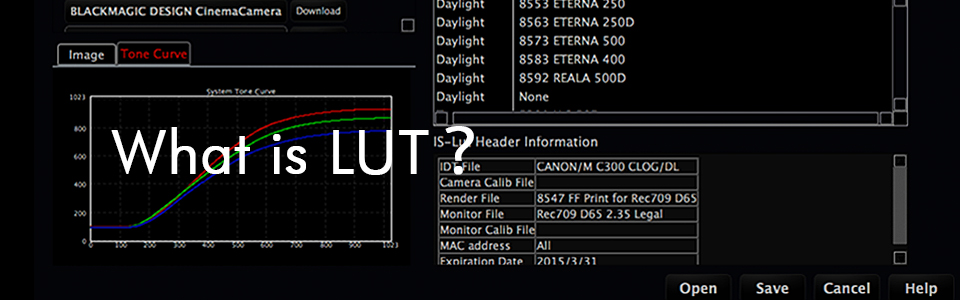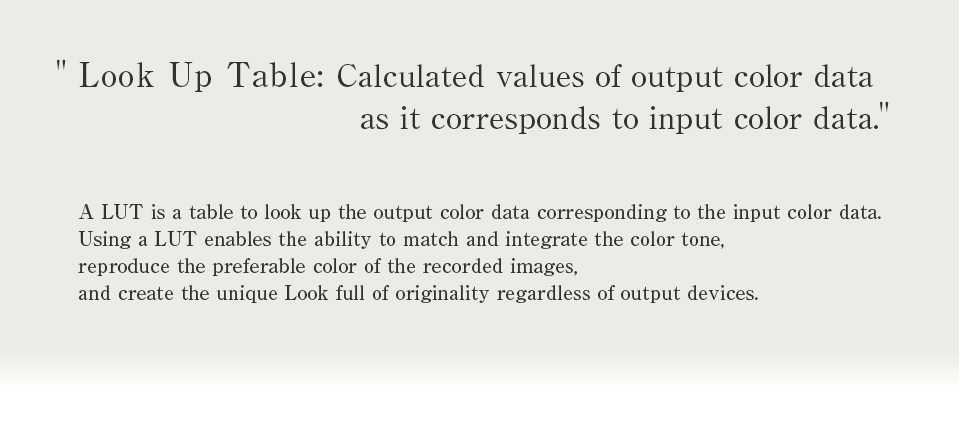##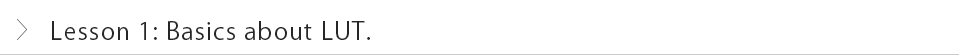### In this first lesson, I would like to explain the "1D LUT" as it is the most important basic concept of all. LUT is called various things: "lut," "Cube," "table," and "L. U. T" depending on the industry. However, it is nothing more than a table that displays the output value corresponding to the input value. When a LUT is used in image processing, its role is to convert a set of three values of RGB to a different RGB set.

Figure 1 displays how to understand a LUT.
As a simple example, let's take a look at a 1D-LUT, which has a single integer input and output, respectively.
X-axis is input and y-axis is output.
For example, if the input value is 80, it will be converted to 50 as output. （A）
In the same way, if the input value is 170, it will be converted to 220 as output.（Ｂ）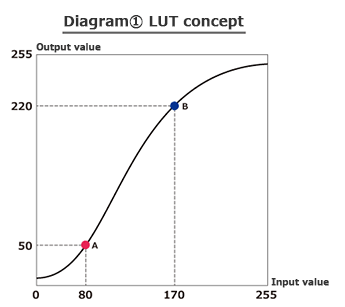If the input is 8bit, it needs 256 output values corresponding to 256 inputs: form 0 to 255.
If we write out all these combinations, it will be like Figure 2
This is what we call a LUT (1D-LUT).
In this example, the left column in Figure 2 shows input values, and the corresponding output values are on the right column.We are basically looking up values on a table, thus the acronym LUT stands for Look Up Table..
Then, let's convert RGB=（80,80,80） using a LUT.
The corresponding input value 80 to the output value is 50; therefore, we will see that the converted output is RGB=(50,50,50).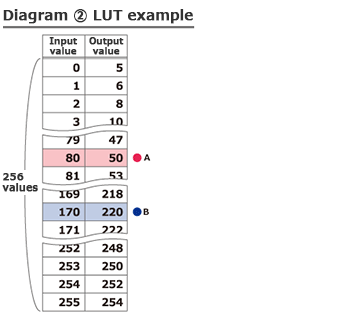Next, let's take a look at the conversion example where each R, G, B value uses a different LUT.
As Figure 3 shows, this uses three LUTs, R-LUT, G-LUT, and B-LUT, respectively.
Using three LUTs,C input RGB=（80,80,80） will be converted to output RGB=(50, 75, 25). You can see that each R, G, B value will be converted to different value.

However!
There are color conversions that cannot be expressed with RGB 1D-LUTs.
For instance, 1D-LUTs cannot execute color conversion that only controls intensity.
In that case, a more complicated "3D-LUT" is necessary.
This scope of this lesson explains 1D-LUTs, so that you will have good basic understanding. What do you think?
Next Time, I am going to explain 3D-LUTs in further details.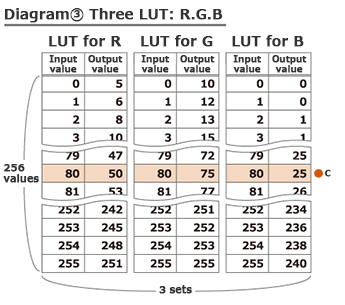From here to the footer.